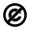# PHYSICS 267: Higher Representations in Physics and Mathematics

PHYSICS 267: Higher Representation Theory in Math and Physics

Logistics:

Office hours in Lyman 227 available during working hours, by appt.

In any given week, a few interested students may (and are encouraged to!) participate in videotaped Q&A discussions on any course-related topics at Bok Center for Learning (Science Center Building)

Prerequisites: In this course, all the methods used are elementary, finite-dimensional linear algebra, but used in very unusual ways. For instance, the course will not use any commutative diagrams.

Grading: Occasional homework assignments posted on Canvas (100%) but grades can be improved by class participation. No exams.

Outline: The course will consist of several big parts, some of which will be developed concurrently.

Part I: Topological quantum field theory (TQFT). Dissection of space will translate into mathematical structures and mathematical theorems. The topics covered will be:

1. TQFT in 1D: projections and orthogonalization
2. TQFT in 2D: algebras with traces, an elementary construction of Verlinde-type invariants for surfaces
3. TQFT in 2D: the computational algorithms. A fast way to block-diagonalize algebras, later used to find automatically all irreducible representations of a finite group or algebra.
4. TQFT in 3D: topological automorphisms of tori viewed as the action of the modular group connected to a representation of Hurwitz innumber theory
5. TQFT in 3D: Wormholes interpreted as the Drinfeld quantum double and its braiding

Part II: Elementary representation theory. We will discover the group representation theory from scratch, on a few simple examples, the cyclic and dihedral groups. We shall concentrate in these explicit cases on interactions between representations, directly computable in these simple examples. We shall describe the representations in the language of wire diagrams in the plane. No previous knowledge of representation theory is needed! We shall extend these techniques explicitly to the spinor group SU(2) and to the interaction between its representations.

Part III: Quantum symmetries of graphs. We show that finite graphs have, besides the classical graph homomorphisms and automorphisms, quantum automorphisms which apply, in the spirit of quantum mechanics, to linear combinations of edges. They use statistical mechanics type computations in plaquette models endowed with Boltzmann weights.

The graphs which have a finite number of quantum automorphisms are the same Dynkin graphs as the graphs describing the crystallography of simple Lie groups (no previous knowledge assumed). This constructs a new, multiplicative quiver theory in which the usual quiver theory viewed as appears in a canonical form.

We show that a finite graph endowed with such plaquettes has a parallel transport like the one of Levi-Civita in general relativity. We compute the flat part of such finite graphs. We connect this structure to invariants found by theoretical physicists to a modular representation of Hurwitz in number theory. We use these quantum graph automorphisms to give a new, direct, and elementary construction from scratch of the crystallographic structure of simple Lie groups.

Thus for instance a quantum version of the symmetries of the dodecahedron, a finite subgroup of SU(2), which we build elementary as quantum symmetries of a graph,indicates precisely how to put together copies of SU(2) to build one of the richest symmetry structures, the Lie group of type E_8.

Part IV: Higher representation theory. The previous theory can be interpreted as a construction of quantum subgroups of SU(2). We now construct, extending these methods, quantum subgroups of SU(3), SU(4), …. Their tensoring graphs provide the higher analogs of the Coxeter-Dynkin diagrams which classify classical crystallography and group representations. These higher  Dynkin diagrams answer a bottle-of-champagne classification question put by the theoretical physicists DiFrancesco and Zuber.

Starting from these graphs, series and exceptionals, we build from scratch a higher (category) version of representation theory: new root lattices, weight lattices, diagonal elements, as well as higher matrices and higher representations. The usual matrix algebra mathematics has implicitly SU(2) as an underlying, or subjacent, group. The higher representation theory uses SU(3), SU(4), …, or any semisimple Lie group as underlying its structure. We describe a new point of view on the Gelfand-Tsetlin explicit construction of representations of SU(N). The new, higher representation theory gives new structure to relations between usual representations. The Racah-Wigner coefficients are for the first time described both combinatorically and crystallographically.

These higher structures may solve the century and a half old problem of finding the full mathematical structure of the octonions, sedenions, etc., by making them part of the higher representations structure.

Conclusions: We finally show that underlying the whole classical and higher representation theory is a discrete form of Gaussian and Riemannian curvature, which allows a very simple geometrical description of these higher representations. Just like the 6j symbols of classical representations are describing interactions between punctures in  1+1 D quantum field theory, the higher representation theory is aimed at describing the interactions between Feynman diagrams in a physical 3+1 D quantum field theory. The higher representations are viewed as the internal symmetry of matter, and thus as the matter itself in this model. As they are described by Riemannian curvature, this would make matter live in the folds of space time. This would provide a concrete mechanism through which matter curves space.

Here is a detailed description of the QFT dictionary between space and algebraic computation which we developed in the course, as it appears in the simplest case.

The precise definitions, which work in all dimensions, are best given after we study a few such examples for motivation.

The following poster illustrates the very rich structure of quantum symmetries of finite graphs, which will be our next topics in the course.This course content is offered under a Public Domain license. Content in this course can be considered under this license unless otherwise noted.